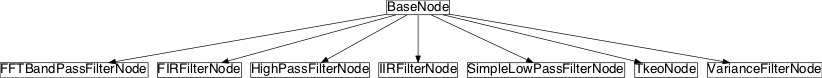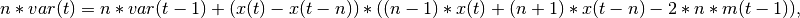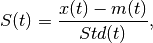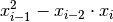# filtering¶

## Module: missions.nodes.preprocessing.filtering¶

Digital filtering of TimeSeries

Inheritance diagram for pySPACE.missions.nodes.preprocessing.filtering:## Class Summary¶

 SimpleLowPassFilterNode(cutoff_frequency[, ...]) Low-pass filtering with the given cutoff frequency using SciPy HighPassFilterNode(cutoff_frequency, taps[, ...]) High-pass filtering with a FIR filter FFTBandPassFilterNode(pass_band[, ...]) Band-pass filtering using a Fourier transform FIRFilterNode(pass_band[, taps, width, ...]) Band-pass or low-pass filtering with a time domain convolution based on a FIR filter kernel IIRFilterNode(pass_band[, pass_band_loss, ...]) Band-pass or low-pass filtering with a direct form IIR filter VarianceFilterNode([width, standardization, ...]) Take the variance as filtered data or standardize with moving variance and mean TkeoNode([selected_channels]) Calculate the energy of a signal with the Teager Kaiser Energy Operator (TKEO) as new signal

## Classes¶

### SimpleLowPassFilterNode¶

class pySPACE.missions.nodes.preprocessing.filtering.SimpleLowPassFilterNode(cutoff_frequency, taps=None, width=None, window='hamming', selected_channels=None, **kwargs)[source]

Low-pass filtering with the given cutoff frequency using SciPy

This node performs low pass filtering with the given cutoff_frequency. It uses a FIR filter whose taps, width, and window can be specified.

Note

Deprecated, because functionality is contained in the other nodes, with much more important features. Use the FIRFilterNode or IIRFilterNode.

Exemplary Call

-
node : SimpleLowPassFilterNode
parameters :
cutoff_frequency : 0.25

Author: Revisited: Jan Hendrik Metzen (jhm@informatik.uni-bremen.de) Hendrik Woehrle (hendrik.woehrle@dfki.de) 2008/08/18 SimpleLowPassFilterNode SimpleLowPassFilter Simple_Low_Pass_Filter TimeSeries

Class Components Summary

 _execute(x) Apply low pass filter to data x and return the result input_types
__init__(cutoff_frequency, taps=None, width=None, window='hamming', selected_channels=None, **kwargs)[source]
_execute(x)[source]

Apply low pass filter to data x and return the result

input_types = ['TimeSeries']

### HighPassFilterNode¶

class pySPACE.missions.nodes.preprocessing.filtering.HighPassFilterNode(cutoff_frequency, taps, width=None, window=('kaiser', 0.5), selected_channels=None, **kwargs)[source]

High-pass filtering with a FIR filter

Parameters

cutoff_frequency:

A frequency in Hz. Frequencies above the cutoff frequency can pass, but below are reduced (attenuated). Recommended cutoff_frequency for EMG preprocessing: 40 Hz

taps:

Number of taps of the filter kernel. Also called filter order. For EMG preprocessing the recommended filter order is 150.

width:

Approximate width of transition region (normalized so that 1 corresponds to pi) for use in kaiser FIR filter design.

(optional, default: None)

window:

Window function to use. See Scipy documentation http://docs.scipy.org/ doc/scipy/reference/generated/scipy.signal.firwin.html#scipy.signal.firwin for possible windows.

(optional, default: (‘kaiser’, 0.5))

selected_channels:

A list of channel names for which the filter should be applied. E.g. the names of the EMG channels.

(optional, default: None)

Exemplary Call

-
node : High_Pass_Filter
parameters :
cutoff_frequency : 0.1
taps : 150
selected_channels : ['C3','C4']

Author: Created: Judith Suttrup 2010/02/10 High_Pass_Filter HighPassFilterNode HighPassFilter TimeSeries

Class Components Summary

 _execute(x) Apply high pass filter to data x and return the result input_types
__init__(cutoff_frequency, taps, width=None, window=('kaiser', 0.5), selected_channels=None, **kwargs)[source]
_execute(x)[source]

Apply high pass filter to data x and return the result

input_types = ['TimeSeries']

### FFTBandPassFilterNode¶

class pySPACE.missions.nodes.preprocessing.filtering.FFTBandPassFilterNode(pass_band, selected_channels=None, **kwargs)[source]

Band-pass filtering using a Fourier transform

This node performs a band-pass filtering for a given pass_band by converting the signal into the frequency domain using an FFT, setting all bands outside the pass band to zero, and going back to the time domain using an IFFT.

Note

Deprecated. Use the FIRFilterNode or IIRFilterNode.

Exemplary Call

-
node : FFTBandPassFilter
parameters :
pass_band : [0.1, 1.0]

Author: Revisited: Jan Hendrik Metzen (jhm@informatik.uni-bremen.de) Hendrik Woehrle (hendrik.woehrle@dfki.de) 2008/08/18 FFTBandPassFilter FFTBandPassFilterNode FFT_Band_Pass_Filter TimeSeries

Class Components Summary

 _execute(x) Apply band pass filter to data x and return the result input_types
__init__(pass_band, selected_channels=None, **kwargs)[source]
_execute(x)[source]

Apply band pass filter to data x and return the result

input_types = ['TimeSeries']

### FIRFilterNode¶

class pySPACE.missions.nodes.preprocessing.filtering.FIRFilterNode(pass_band, taps=33, width=None, window='hamming', skip=0, comp_type='normal', time_shift='middle', selected_channels=None, **kwargs)[source]

Band-pass or low-pass filtering with a time domain convolution based on a FIR filter kernel

This node performs a finite impulse response filtering for a given pass_band by applying a time domain convolution with a FIR filter kernel.

Parameters

pass_band: The pass band. Tuple for band pass, single value for low pass filtering. Number of taps of the filter kernel (i.e. order-1) (optional, default: 33) Approximate width of transition region (normalized so that 1 corresponds to pi) for use in kaiser FIR filter design. (optional, default: None) Window function to use. See scipy doc for possible windows. (optional, default: ‘hamming’) Type of computation, e.g. ‘normal’: the computations are performed using scipy ‘parallel’: the computations are performed using the adappt module (optional, default: ‘normal’) Number of values that are skipped during convolution, for e.g. in decimation. Needs the comp_type set to ‘parallel’. (optional, default: 0) Normally, the convolution is performed as follows: If a signal of length N is convolved with a signal of length M, the result has the length N+M-1. Scipy picks the first N values to assure, that the resulting signal is of valid length. If time_shift is set to ‘normal, the filter behaves as stated above. If time_shift is set to ‘middle’, the values of the interval [M/2,N+M/2-1] are picked. If time_shift is set to ‘end’, the values of the interval [(N+M-1)/2,N+M-1] are picked. If time_shift is set to ‘stream’, a block-wise computation is performed, i.e. all incoming time series objects are assumed to be adjacent sub-blocks of a larger data stream. Therefore, the internal filter state is preserved between different executions of the filter. (optional, default: False)

Exemplary Call

-
node : FIRBandPassFilter
parameters :
pass_band : [0.4]
comp_type : "normal"
window : "hamming"
taps : 3
skip : 0

Author: POSSIBLE NODE NAMES: Hendrik Woehrle (hendrik.woehrle@dfki.de) FIRFilterNode FIRFilter FIRLowPassFilter FIRBandPassFilter BandPassFilter LowPassFilter TimeSeries

Class Components Summary

 __setstate__(sdict) Restore object from its pickled state _execute(data) Apply filter to data and return the result calc_filter_kernel(data) Calculate filter kernel initialize_data_dependencies(data) Initialize several data dependent buffer variables input_types
__init__(pass_band, taps=33, width=None, window='hamming', skip=0, comp_type='normal', time_shift='middle', selected_channels=None, **kwargs)[source]
initialize_data_dependencies(data)[source]

Initialize several data dependent buffer variables and data items

calc_filter_kernel(data)[source]

Calculate filter kernel

_execute(data)[source]

Apply filter to data and return the result

__setstate__(sdict)[source]

Restore object from its pickled state

input_types = ['TimeSeries']

### IIRFilterNode¶

class pySPACE.missions.nodes.preprocessing.filtering.IIRFilterNode(pass_band, pass_band_loss=0.5, stop_band_rifle=60, ftype='ellip', selected_channels=None, comp_type='normal', **kwargs)[source]

Band-pass or low-pass filtering with a direct form IIR filter

Parameters

pass_band: pass_band_loss: The pass band. Tuple for band pass, single value for low pass filtering. Allowed pass band loss in dB. (optional, default: 0.5) Allowed remaining stop band rifle in dB. (optional, default: 60) Type of used filter, e.g. elliptic or butterworth. See scipy.signal.filter_design.iirdesign for further information. selected_channels Type of computation, e.g. ‘normal’ (optional, default: ‘normal’)

Exemplary Call

-
node : IIRBandPassFilter
parameters :
pass_band : [0.4]
comp_type : "normal"
window : "hamming"
taps : 3
skip : 0

Author: POSSIBLE NODE NAMES: Hendrik Woehrle (hendrik.woehrle@dfki.de) IIRLowPassFilter IIRBandPassFilter IIRFilter IIRFilterNode TimeSeries

Class Components Summary

 _execute(data) Apply filter to data and return the result .. calc_filter_kernel(data) Calculate filter kernel input_types
__init__(pass_band, pass_band_loss=0.5, stop_band_rifle=60, ftype='ellip', selected_channels=None, comp_type='normal', **kwargs)[source]
calc_filter_kernel(data)[source]

Calculate filter kernel

_execute(data)[source]

Apply filter to data and return the result .. todo:: check if other view is needed here please

input_types = ['TimeSeries']

### VarianceFilterNode¶

class pySPACE.missions.nodes.preprocessing.filtering.VarianceFilterNode(width=50, standardization=False, normalize=False, lenNormalize=1, **kwargs)[source]

Take the variance as filtered data or standardize with moving variance and mean

This node can perform a low-pass filtering using the variance, for example used to enhance the SNR of raw EMG Signals, or calculates a standardization with the variance.

Filter:

The variance is calculated for each sample at time point t using the following formula:where:
• n is the width of the “filter”, number of samples used for calculating the variance
• Var(t) is the variance as time point t
• x(t) is the sample at time point t
• m(t) is the mean at time point t
Standardization:

The standardization is calculated for each sample at time point t using the following formula:where:
• S(t) = is the standardization for the sample at time point t
• x(t) is the sample at time point t
• m(t) is the mean at time point t
• Std(t) is the standard deviation at time point t

The standard deviation is calculated using the formula for the variance explained above, followed by a applying the normal square root

Parameters

width: standardization: Size of the window used to calculate the variance, the higher the value the smoother is the resulting signal. The value is given in ms. (optional, default: 50) Flag which indicates if the filter should simply calculate the variance for a given size (False), or if a standardization should be calculated, meaning the mean for the given size is subtracted from the sample followed by a division by the variance. (optional, default: False)

Exemplary Call

-
node : VarianceFilter
parameters :
width : 2000
standardization : False

Author: Created: Marc Tabie (mtabie@informatik.uni-bremen.de) 2012/05/02 VarianceFilter VarianceFilterNode TimeSeries

Class Components Summary

 _execute(data) input_types standardisation(outData, inData, ringbuffer, ...) variance(outData, inData, ringbuffer, ...)
__init__(width=50, standardization=False, normalize=False, lenNormalize=1, **kwargs)[source]
_execute(data)[source]
variance(outData, inData, ringbuffer, variables, width, index)[source]
standardisation(outData, inData, ringbuffer, variables, width, index)[source]
input_types = ['TimeSeries']

### TkeoNode¶

class pySPACE.missions.nodes.preprocessing.filtering.TkeoNode(selected_channels=None, **kwargs)[source]

Calculate the energy of a signal with the Teager Kaiser Energy Operator (TKEO) as new signal

This is a quadratic filter with the formula:The formula is taken from the following publication:

Kaiser J. F. (1990)
On a simple algorithm to calculate 'energy' of a signal.
In Proceedings:
International Conference on Acoustics, Speech, and Signal Processing (ICASSP-90)
Pages 381-384
(http://ieeexplore.ieee.org/stamp/stamp.jsp?tp=&arnumber=115702)


For processing EMG-Data a high pass filter at 20 Hz before the node and a low pass filter at 50 Hz after the node is recommended.

Parameters

selected_channels:

A list of channel names the algorithm should work with. I.e. the EMG channel names. If this parameter is not specified, all channels are used.

(optional, default: None)

Exemplary Call

-
node : TKEO
parameters :
selected_channels : ["C3", "C4"]

Author: Created: Marc Tabie (mtabie@informatik.uni-bremen.de) 2012/05/02 Tkeo TkeoNode TKEO TimeSeries

Class Components Summary

 _execute(x) Compute the energy of the given signal x using the TKEO input_types
__init__(selected_channels=None, **kwargs)[source]
_execute(x)[source]

Compute the energy of the given signal x using the TKEO

input_types = ['TimeSeries']